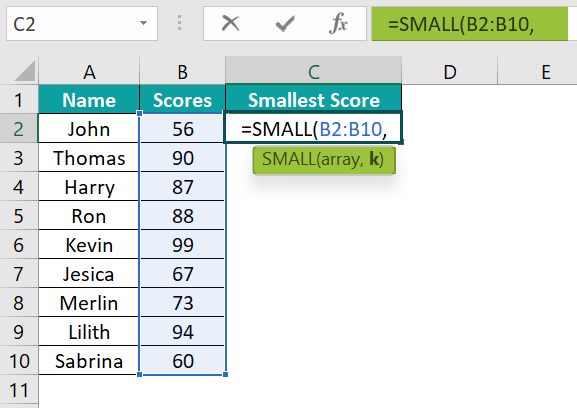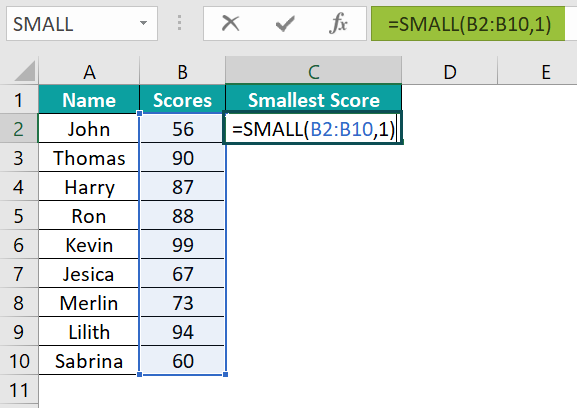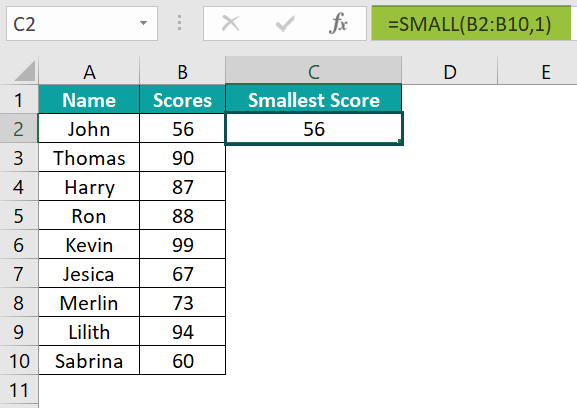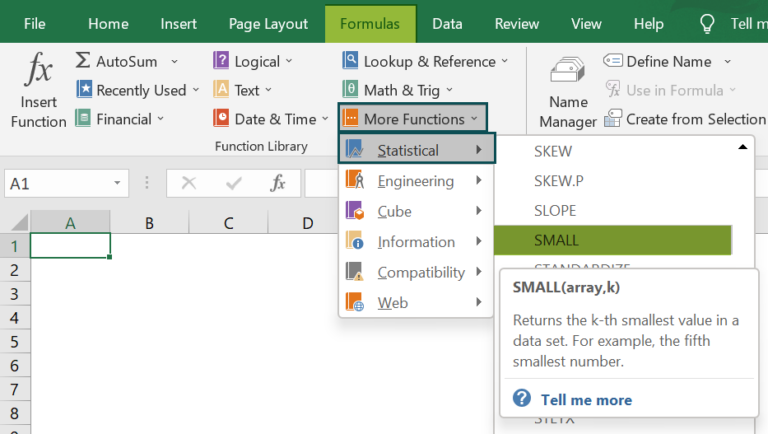# SMALL Function In ExcelArticle byExcelMojo Team## What Is the SMALL Function In Excel?

The SMALL function in Excel finds the smallest number from the dataset or an array of values. This function returns the “kth” i.e., the smallest numerical value from the selected values. The SMALL Function in Excel is a built-in function of the “Statistical” Function, so we can enter it as a formula or insert it from the Function Library.

For example, we will calculate the smallest number using the SMALL Function.

Select cell B2, enter the formula =SMALL(A2:A3,1), and press the “Enter” key.

The output is “10”, as shown above. In column A values, the smallest number is returned as the output.

###### Key Takeaways
• Instead of sorting the values in a dataset into ascending order, we use the SMALL Function in Excel to fetch the smallest value.
• If duplicate values exist, the function will consider the first value as the smallest.
• The “k” value should be numeric, or it will return the #VALUE! error.
• The value of the array should not be empty.
• In the selected cell range, the SMALL Function in Excel ignores the text values
•  If there are any errors in the selected cells, we will get the #DIV/0! error.

### SMALL() Excel Formula

The Syntax of the SMALL Excel Formula is,

The arguments of the SMALL Excel Formula are,

• array: It is a mandatory argument. It is the range or array from which we want the function to return the kth smallest value.
• k: It is a mandatory argument. It is an integer that specifies the position from the value, i.e., the smallest “kth” position.

### How To Use SMALL Excel Function?

We can use the SMALL Function in Excel in 2 methods, namely,

1. Access from the Excel ribbon.
2. Enter the worksheet manually.

#### Method #1 – Access from the Excel ribbon

Choose the empty cell for the result 🡪 select the “Formulas” tab 🡪 go to the “Function Library” group 🡪 click the “More Functions” drop-down 🡪 click the “Statistical” option right arrow 🡪 select the “SMALL” function, as shown below.

The “Function Arguments” window pops up, instantly, inserting the SMALL function in Excel. First, enter the value in the “array” and “k” fields. Then, click “OK”, as shown below.

#### Method #2 – Enter in the worksheet manually

1. Select an empty cell for the output.
2. Type =SMALL (in the selected cell). [Alternatively, type “=S” or “=SM” and double-click the SMALL function from the list of suggestions shown by Excel.]
3. Enter the arguments as excel cell references or direct values.
4. Close the parenthesis and press the “Enter” key.

Let us take a basic example where we will find the smallest score using SMALL in Excel from the student scores in a class.

In the table, the data is,

• Column A contains the Name.
• Column B contains the Scores.
• Column C displays the Output.

The steps to find the smallest value using SMALL Function in Excel are:

1. Select cell C2 and enter the formula =SMALL(B2:B10,2. Enter the value of ‘k’ as 1, i.e., the first smallest number in the array, in continuation of the formula, and close the bracket. The complete formula is =SMALL(B2:B10,1).3. Press the “Enter” key. The smallest score is ‘56’ as shown below.### Examples

We will understand the SMALL function in Excel with some advanced scenarios.

#### Example #1

We will find the 1st and 2nd smallest salaries of the employees from the XYZ company using the SMALL function in Excel.

In the table, the data is,

• Column A contains the Name.
• Column B contains the Salary.

The steps to detect the smallest salary using the SMALL Function in Excel are:

Step 1: First, select cell B6 and enter the formula =SMALL(B2:B5,1).

Step 2: Press the “Enter” key. The output is shown below.

Step 3: Next, select cell B7 and enter the formula =SMALL(B2:B5,2).

Step 4: Press the “Enter” key. The 1st and the 2nd smallest salaries are shown in the image below.

#### Example #2

We will find the smallest value from the table array using the SMALL function in Excel.

In the table, the data is,

• Column A contains the Values.

The steps to find the smallest value by the SMALL Function in Excel are:

Step 1: Select cell B2 and enter the formula =SMALL(A2:A10,1).

Step 2: Press the “Enter” key. The output is ‘0.56’ as shown below.

#### Example #3

We will find the least cost using the SMALL function in Excel from the stocks of the items of the garments shop.

In the table, the data is,

• Column A contains the Items.
• Column B contains the Stock.

The steps to detect the smallest value by the SMALL Function are as follows:

Step 1: Select cell B6 and enter the formula =SMALL(B2:B5,1).

Step 2: Press the “Enter” key. The output is ‘100’, as shown below.

### Important Things to Note

• The SMALL Function in Excel is under the Statistical Function category and returns a numeric value based on its position in a selected list of values.
• We will get the #NUM! error:
• If the array used is empty.
• If the value of ‘k’ is less than the numerical value is 1 or greater than the values or count in the selected array.
• We will get the #VALUE! error if the value is non-numeric.
• The #NA error occurs when one of the values in the selected array is an error, i.e., “#NA”.

1. What does the SMALL function do in Excel?

The SMALL Function in Excel categorized under the Statistical function returns numeric values based on their position in a list, ranked by value, like if the values are sorted in ascending order. It calculates and returns the ‘k’, i.e., the smallest value in a dataset, depending on the kth-value input.
The formula of the SMALL Function in Excel is =SMALL(array,k)

2. Why is the SMALL function in Excel not working?

The SMALL function in Excel may not work for the following reasons,
● We will get the #NUM! error,
● If the array used is empty.
● If the value of ‘k’ is less than the numerical value is 1 or greater than the values or count in the selected array.
● The #VALUE! error occurs if the value is non-numeric.

3. Where is the SMALL function in Excel?

We can insert the SMALL function in Excel as follows:
First, choose the empty cell for the result 🡪 select the “Formulas” tab 🡪 go to the “Function Library” group 🡪 click the “More Functions” drop-down 🡪 click the “Statistical” option right arrow 🡪 select the “SMALL” function, as shown below.